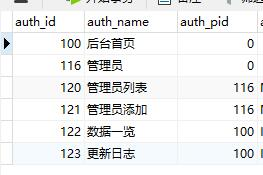# PHP实现无限极分类的两种方式，递归和引用

https://blog.csdn.net/falcom_fans/article/details/75579663### 国民级算法——递归

function getTree($arr,$pid=0,$level=0) { static$list = [];
foreach ($arr as$key => $value) { if ($value["auth_pid"] == $pid) {$value["level"] = $level;$list[] = $value; unset($arr[$key]); //删除已经排好的数据为了减少遍历的次数，当然递归本身就很费神就是了 getTree($arr,$value["id"],$level+1);
}
}
return $list; } 基本也没啥好说的，这里返回去的是一个已经排序好的一维数组，展示的时候直接遍历就好，加入level字段是为了展示的时候，如果需要缩进，可以有个依据 ### 巧妙的引用算法 上面的递归原理通俗易懂，但是总所周知的原因，递归对资源的消耗是非常大的，实际执行起来效率也很低，所以有了下面的通过引用算法 function generateTree($data){
$items = array(); foreach($data as $v){$items[$v['auth_id']] =$v;
}
$tree = array(); foreach($items as $k =>$item){
if(isset($items[$item['auth_pid']])){
$items[$item['auth_pid']]['son'][] = &$items[$k];
}else{
$tree[] = &$items[$k]; } } return$tree;
}

   $items = array(); foreach($data as $v){$items[$v['auth_id']] =$v;
}

Array
(
 => Array
(
[auth_id] => 100
[auth_name] => 后台首页
[auth_pid] => 0
)

 => Array
(
[auth_id] => 116
[auth_name] => 管理员
[auth_pid] => 0
)

 => Array
(
[auth_id] => 120
[auth_name] => 管理员列表
[auth_pid] => 116
)

 => Array
(
[auth_id] => 121
[auth_name] => 管理员添加
[auth_pid] => 116
)

 => Array
(
[auth_id] => 122
[auth_name] => 数据一览
[auth_pid] => 100
)

 => Array
(
[auth_id] => 123
[auth_name] => 更新日志
[auth_pid] => 100
)
)

 $tree = array(); foreach($items as $k =>$item){
if(isset($items[$item['auth_pid']])){
$items[$item['auth_pid']]['son'][] = &$items[$k];
}else{
$tree[] = &$items[$k]; } } return$tree;

$k = 100;$item = Array
(
[auth_id] => 100
[auth_name] => 后台首页
[auth_pid] => 0
)
$items[$item['auth_pid']] = $items; //不存在键值为0的数组元素，证明是顶级分类 isset($items[$item['auth_pid']]) = false;$tree[] = &$items[$k];

$k = 120;$item = Array
(
[auth_id] => 120
[auth_name] => 管理员列表
[auth_pid] => 116
)
$items[$item['auth_pid']] = $items; isset($items[$item['auth_pid']]) = true; //存在键值为116的数组元素，证明这个元素是键值116元素的子分类$items[$item['auth_pid']]['son'][] = &$items[$k];//给键值为116的数组元素增加一个son键，并将当前遍历的这个元素赋值给这个键 这里也是采取了引用，还是那个原因，因为当前遍历的元素很有可能还有子分类，当有子分类的时候，按照这个算法，他自己还要增加son这个键，所以采用引用赋值的方式，可以保证自己的结构是完美的 整体来看这个算法，如果加上两句输出来看一下 $tree = array();
foreach($items as$k => $item){ if(isset($items[$item['auth_pid']])){$items[$item['auth_pid']]['son'][] = &$items[$k]; echo "1111<br>"; }else{ echo "2222<br>";$tree[] = &$items[$k];
}
}

2222
2222
1111
1111
1111
1111


Array
(
 => Array
(
[auth_id] => 100
[auth_name] => 后台首页
[auth_pid] => 0
[son] => Array
(
 => Array
(
[auth_id] => 122
[auth_name] => 数据一览
[auth_pid] => 100
)
 => Array
(
[auth_id] => 123
[auth_name] => 更新日志
[auth_pid] => 100
)
)
)
 => Array
(
[auth_id] => 116
[auth_name] => 管理员
[auth_pid] => 0
[son] => Array
(
 => Array
(
[auth_id] => 120
[auth_name] => 管理员列表
[auth_pid] => 116
)
 => Array
(
[auth_id] => 121
[auth_name] => 管理员添加
[auth_pid] => 116
)
)
)
)


06-051727
08-21308309-28463
09-267066
11-032万+
06-152134
05-241万+
02-195287
12-2987